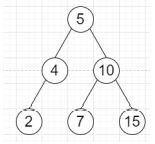# Program to check whether all leaves are at same level or not in Python

Suppose we have a binary tree; we have to check whether all leaves are at the same level or not.

So, if the input is likethen the output will be True

To solve this, we will follow these steps −

• Define a function dfs() . This will take root, d

• if root is not null, then

• if left of root is null and right of root is null, then

• insert d at the end of depth

• otherwise,

• dfs(left of root, d + 1)

• dfs(right of root, d + 1)

• From the main method, do the following −

• depth := a new list

• dfs(root, 0)

• return true when depth has only one value

Let us see the following implementation to get better understanding −

## Example

Live Demo

class TreeNode:
def __init__(self, value):
self.val = value
self.left = None
self.right = None

class Solution:
def solve(self, root):
self.depth = []
self.dfs(root, 0)
return len(set(self.depth)) == 1
def dfs(self, root, depth):
if root:
if not root.left and not root.right:
self.depth.append(depth)
else:
self.dfs(root.left, depth + 1)
self.dfs(root.right, depth + 1)
ob = Solution()
root = TreeNode(5)
root.left = TreeNode(4)
root.left.left = TreeNode(2)
root.right = TreeNode(10)
root.right.left = TreeNode(7)
root.right.right = TreeNode(15)
print(ob.solve(root))

## Input

root = TreeNode(5)
root.left = TreeNode(4)
root.left.left = TreeNode(2)
root.right = TreeNode(10)
root.right.left = TreeNode(7)
root.right.right = TreeNode(15)

## Output

True